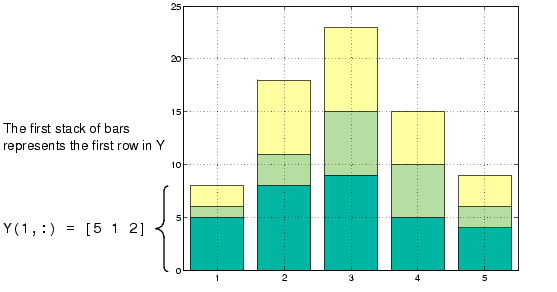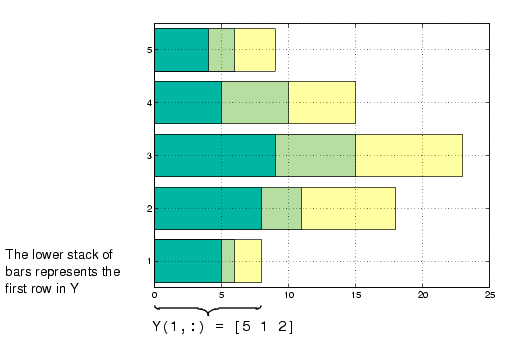GraphicsStacked Bar Graphs to Show Contributing Amounts

Bar graphs can show how elements in the same row of a matrix contribute to the sum of all elements in the row. These types of bar graphs are referred to as stacked bar graphs.

Stacked bar graphs display one bar per row of a matrix. The bars are divided into n segments, where n is the number of columns in the matrix. For vertical bar graphs, the height of each bar equals the sum of the elements in the row. Each segment is equal to the value of its respective element.

Redefining Y

• ```Y = [5 1 2
8 3 7
9 6 8
5 5 5
4 2 3];
```

Create stacked bar graphs using the optional `'stack'` argument. For example,

• ```bar(Y,'stack')
grid on
set(gca,'Layer','top') % display gridlines on top of graph
```

creates a 2-D stacked bar graph, where all elements in a row correspond to the same x location.Horizontal Bar Graphs

For horizontal bar graphs, the length of each bar equals the sum of the elements in the row. The length of each segment is equal to the value of its respective element.

• ```barh(Y,'stack')
grid on
set(gca,'Layer','top') % Display gridlines on top of graph
```Types of Bar Graphs Specifying X-Axis Data© 1994-2005 The MathWorks, Inc.